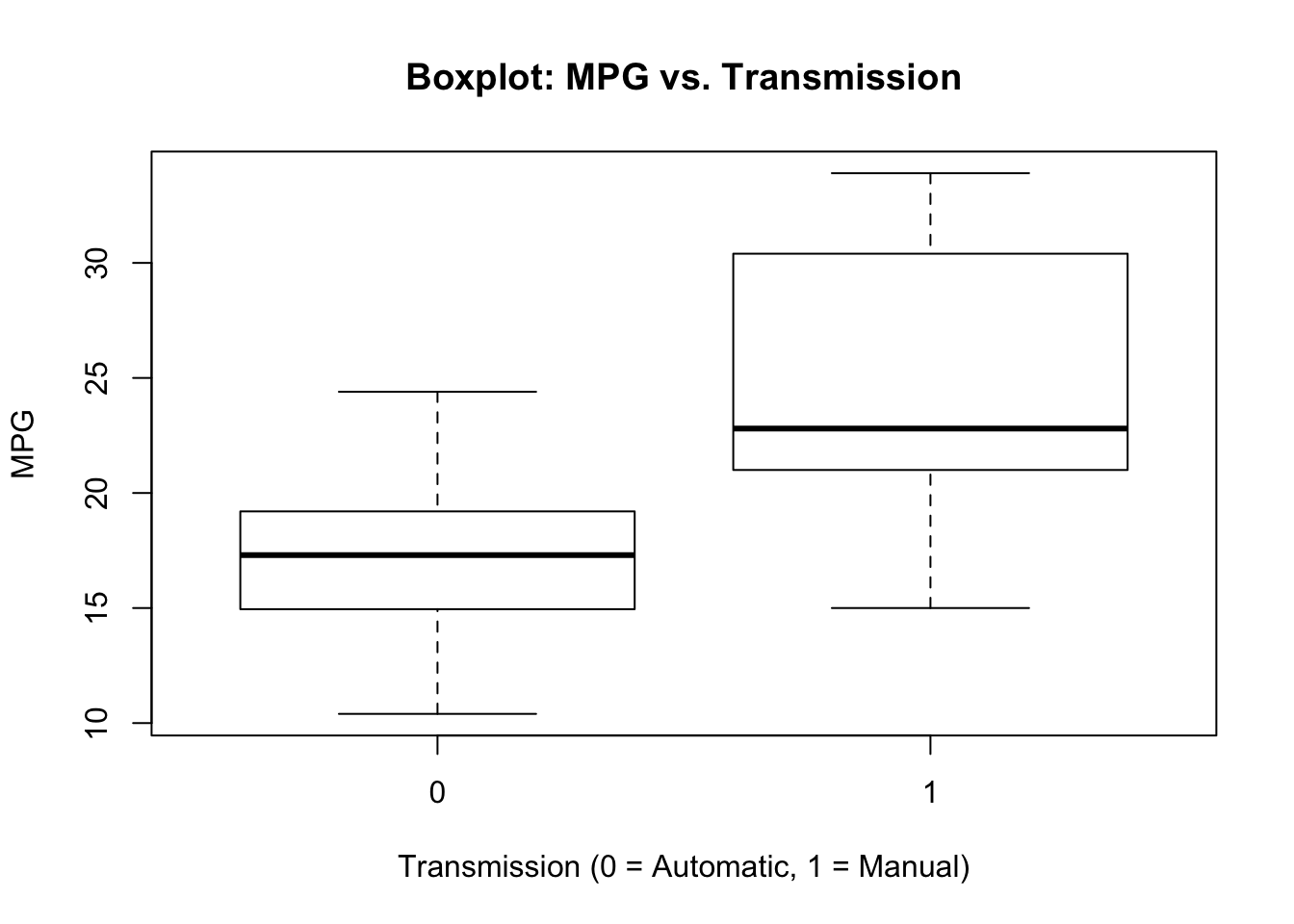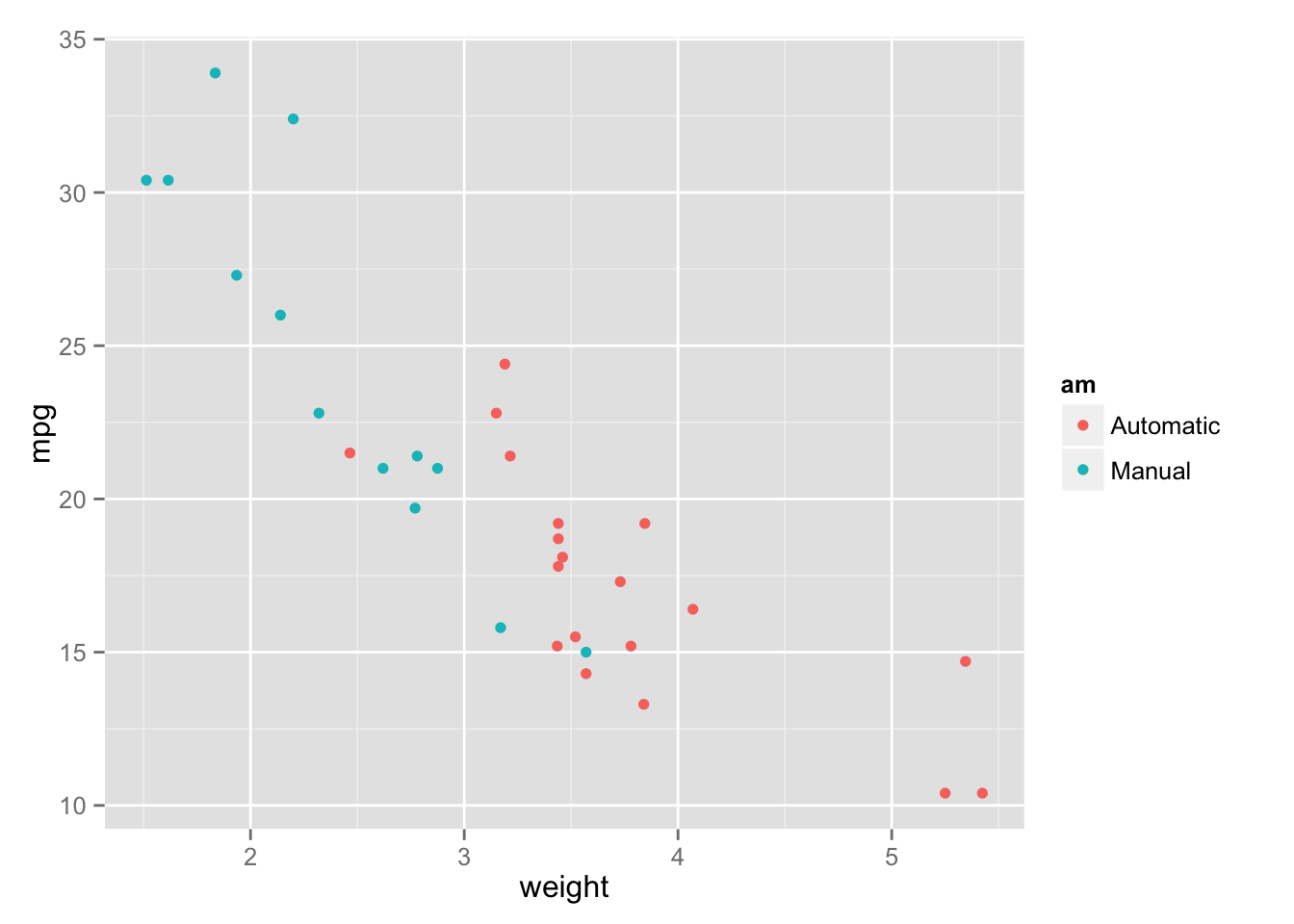This report provides basic summary and exploratory data analysis of the mtcars dataset located in the R datasets package. We focus in particular on determining whether automatic or manual transmission is better for fuel efficiency, and then quantify the difference between the two. We use regression models and exploratory data analyses to examine how automatic and manual transmissions features affect fuel efficiency as measured in miles per gallon. We show that cars with manual transmission have greater fuel efficiency than those with an automatic transmission. We then fit several linear regression models and select the one that explains the most variance in fuel efficiency and quantify the difference between the two types of transmissions in this model. We focus in particular on the role that weight of the car plays in explaining the difference between manual and automatic transmission fuel efficiency.

## Exploratory Data Analysis

The following code loads the dataset mtcars and examines the variables and dimensions of the dataset. It shows that there are 10 aspects of automobile design and a measure of miles per gallon for 32 automobiles. We also adapt the \(am\) variable to a factor to prepare for subsequent analyses.

This step involves basic exploratory analyses. The boxplot (see Appendix) appears to show that manual transmission has higher MPG than automatic. While there was not space in the appendix to include displays, further examination of the data also show that there appear to be high or moderate correlations between other variables in the dataset, such as weight (wt) and horsepower (hp).

## Inference

Assuming that MPG has a normal distribution, we use a two sample t-test to examine the null hypothesis that the MPG of manual and automatic transmissions are the same. With a p-value of .000137, we reject the null hypothesis and conclude that the MPG of the automatic and manual transimissions are different.

``##  0.001373638``
``````## mean in group 0 mean in group 1
##        17.14737        24.39231``````

## Regression Models

The first part of regression analysis involves regressing MPG on all variables.

``````##                Estimate  Std. Error    t value   Pr(>|t|)
## (Intercept) 12.30337416 18.71788443  0.6573058 0.51812440
## cyl         -0.11144048  1.04502336 -0.1066392 0.91608738
## disp         0.01333524  0.01785750  0.7467585 0.46348865
## hp          -0.02148212  0.02176858 -0.9868407 0.33495531
## drat         0.78711097  1.63537307  0.4813036 0.63527790
## wt          -3.71530393  1.89441430 -1.9611887 0.06325215
## qsec         0.82104075  0.73084480  1.1234133 0.27394127
## vs           0.31776281  2.10450861  0.1509915 0.88142347
## am1          2.52022689  2.05665055  1.2254035 0.23398971
## gear         0.65541302  1.49325996  0.4389142 0.66520643
## carb        -0.19941925  0.82875250 -0.2406258 0.81217871``````

The results are interesting: the model shows a residual error of 2.833 with 15 degrees of freedom, and the model explains nearly 78% of the variance in MPG, yet none of the coefficients are significant (at the .05 level). The next step is to use backward model selection.

``````newmodel<-step(full, k=log(nrow(mtcars)))
summary(newmodel)``````

This process shows the best model as regressing MPG on “wt”“,”qsec“” and “am”. The residual error is 2.459 with 28 degrees of freedom and accounts for about 83.4% of the variance of MPG. In this case, all of the coefficients are significant (at the .05 level). The scatterplot of MPG versus weight varying transmission shows a possible interaction between the “wt” and “am” variables. This makes sense as automatic cars probably weigh more than manual cars. The subsequent model is:

``````IntModel<-lm(mpg~wt+qsec+am+wt:am, data=mtcars)
summary(IntModel)``````

The interaction model has the residual error 2.084 with 27 degrees of freedom. The model accounts for about 88% of the variance in MPG and all coefficients are significant at the .05 level. Now that we have fit the best model, we examine how much we improve from the most simple model where transmission is the only predictor variable.

This model shows that a car with automatic transmission on average has 17.147 MPG while a manual transmission increases MPG by 7.245 MPG to 24.392 MPG. The model has 4.902 residual error with 30 degrees of freedom. The model can explain about 34% of the variance in MPG, which suggests other variables would be useful in the model.

We compare the models up to this point and select the best model from earlier and find the interaction model has the highest adjusted R-squared value of the models. This shows that holding constant weight and quarter mile tim,cars with manual transmission have 14.079 -4.141(weight) more MPG than cars with automatic transmission. This means that a manual car weighing 3000 pounds will have about 1.656 more MPG than an automatic car with the same weight.

``summary(IntModel)\$coef``
``````##              Estimate Std. Error   t value     Pr(>|t|)
## (Intercept)  9.723053  5.8990407  1.648243 0.1108925394
## wt          -2.936531  0.6660253 -4.409038 0.0001488947
## qsec         1.016974  0.2520152  4.035366 0.0004030165
## am1         14.079428  3.4352512  4.098515 0.0003408693
## wt:am1      -4.141376  1.1968119 -3.460340 0.0018085763``````

## Residual Analysis and Betas Diagnostic

According to the residual plots (see Appendix), the following are true. First, the Residuals vs. Fitted plot supports the accuracy of the independence assumption.Second, the Normal Q-Q plot indicates that the residuals are normally distributed.Third, the Scale-Location plot confirms the constant variance.Fourth, the Residuals vs. Leverage appears to show no outliers.The Dfbetas (see below) also appears to show that there are no outliers. These tests show the assumptions made are reasonable for the analyses performed.

## Appendix

### Boxplot of MPG and Transmission### Scatterplot of MPG and Weight by Transmission